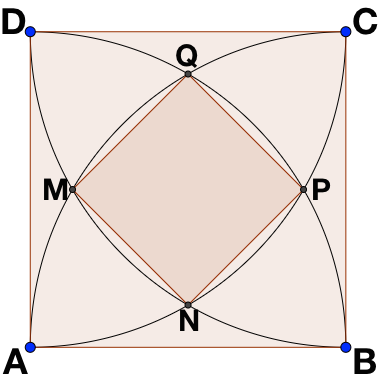# Square Formed From Intersecting Arcs

Geometry Level 2It is given that the area of square $ABCD$ is $6 + 3 \sqrt{3}$. Find the area of square $MNPQ$.

Note: Each square's vertex is a circumcentre of a circular arc.

×

Problem Loading...

Note Loading...

Set Loading...